### Trigonometry Class 10th Mathematics AP Board Solution

##### Question 1.Evaluate the following :A. (1 + tanθ + secθ)(1 + cotθ – cosecθ)Answer:We have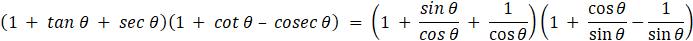⇒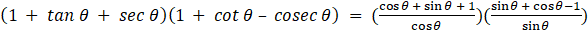⇒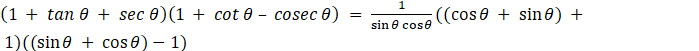⇒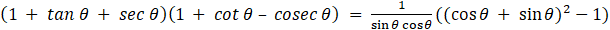[∵, (a + b)(a – b) = a2 – b2]⇒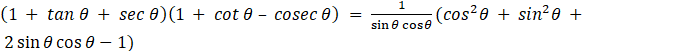[∵, (a + b)2 = a2 + b2 + 2ab]⇒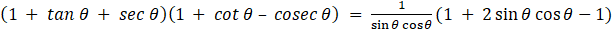[∵, sin2 θ + cos2 θ = 1]⇒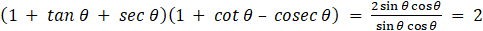Thus, (1 + tan θ + sec θ)(1 + cot θ – cosec θ) = 2.Question 2.Evaluate the following :B. (sinθ + cosθ)2 + (sinθ - cosθ)2Answer:We have(sin θ + cos θ)2 + (sin θ – cos θ)2 = ((sin θ + cos θ) + (sin θ – cos θ))2 – 2(sin θ + cos θ)(sin θ – cos θ) [∵, a2 + b2 = (a + b)2 – 2ab]⇒ (sin θ + cos θ)2 + (sin θ – cos θ)2 = (sin θ + cos θ + sin θ – cos θ)2 – 2(sin2 θ – cos2 θ)[∵, (a + b)(a – b) = a2 – b2]⇒ (sin θ + cos θ)2 + (sin θ – cos θ)2 = (2 sin θ)2 – 2 sin2 θ + 2 cos2 θ⇒ (sin θ + cos θ)2 + (sin θ – cos θ)2 = 4 sin2 θ – 2 sin2 θ + 2 cos2 θ⇒ (sin θ + cos θ)2 + (sin θ – cos θ)2 = 2 sin2 θ + 2 cos2θ⇒ (sin θ + cos θ)2 + (sin θ – cos θ)2 = 2 (sin2 θ + cos2 θ)⇒ (sin θ + cos θ)2 + (sin θ – cos θ)2 = 2 [∵, sin2 θ + cos2 θ = 1]Thus, (sin θ + cos θ)2 + (sin θ – cos θ)2 = 2.Question 3.Evaluate the following :C. (sec2θ – 1) (cosec2θ – 1)Answer:We have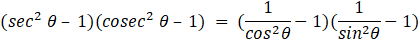⇒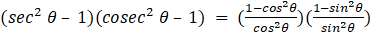⇒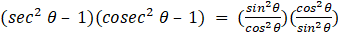[∵, (1 – cos2 θ) = sin2 θ & (1 – sin2 θ = cos2 θ]⇒ (sec2 θ – 1)(cosec2 θ – 1) = 1Thus, (sec2 θ – 1)(cosec2 θ – 1) = 1.Question 4.Show that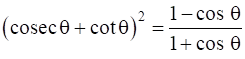Answer:Using trigonometric identities,cosec θ = 1/sin θ & cot θ = cos θ/sin θLHS = (cosec θ – cot θ)2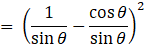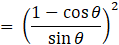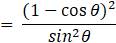As we know, sin2 θ = 1 – cos2 θAnd (1 – cos2 θ) = (1 + cos θ)(1 – cos θ) [by (a2 – b2) = (a + b)(a – b)]⇒ sin2 θ = (1 + cos θ)(1 – cos θ) …(i)So, using equation (i),LHS = (cosec θ – cot θ)2 =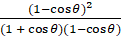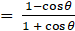= RHSHence, we have got(cosec θ – cot θ)2 =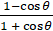.Question 5.Show that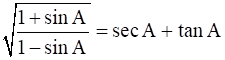Answer:Using trigonometric identity,sin2 A + cos2 A = 1⇒ cos2 A = 1 – sin2 ATake Left hand side:LHS =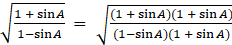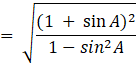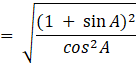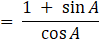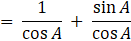= sec A + tan A = RHSThus,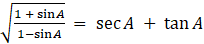.Question 6.Show that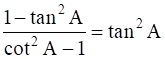Answer:Using trigonometric identity, we havecot A = 1/tan ATake left hand side,LHS =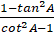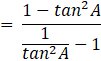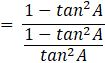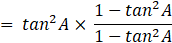= tan2 A = RHSThus,= tan2 A.Question 7.Show that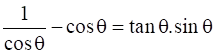Answer:Take left hand side of the given question:LHS = 1/cos θ – cos θ= (1 – cos2 θ)/cos θ= sin2 θ/cos θ [∵, sin2 θ + cos2 θ = 1 ⇒ sin2 θ = 1 – cos2 θ]= sin θ × sin θ/cos θ= sin θ × tan θ [∵, tan θ = sin θ/cos θ]= tan θ sin θ = RHSThus,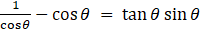.Question 8.Simplify secA(1 – sinA)(secA + tanA)Answer:By trigonometric identities, sec A = 1/cos A & tan A = sin A/cos AUsing these identities, we havesec A (1 – sin A)(sec A + tan A) =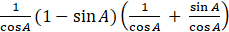⇒ sec A (1 – sin A)(sec A + tan A) =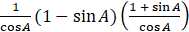⇒ sec A (1 – sin A)(sec A + tan A) =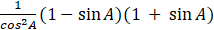⇒ sec A (1 – sin A)(sec A + tan A) =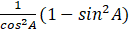⇒ sec A (1 – sin A)(sec A + tan A) =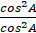[∵, sin2 A + cos2 A = 1 ⇒ cos2 A = 1 – sin2 A]⇒ sec A (1 – sin A)(sec A + tan A) = 1Thus, sec A (1 – sin A)(sec A + tan A) = 1.Question 9.Prove that (sin A + cosec A)2 + (cos A + sec A)2 = 7 + tan2 A + cot2 AAnswer:Take left hand side of the given equation:LHS = (sin A + cosec A)2 + (cos A + sec A)2Expanding the squares by formula: (a + b)2 = a2 + b2 + 2ab= sin2 A + cosec2 A + 2 sin A cosec A + cos2 A + sec2 A + 2 cos A sec ARearranging the terms, we get,= (sin2 A + cos2 A) + 2 sin A cosec A + 2 cos A sec A + cosec2 A + sec2 Awe know that,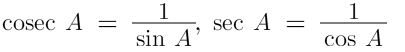=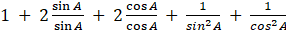=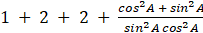= 5 + 1/(sin2 A cos2 A) …(i)Now, take right hand side of the equation:RHS = 7 + tan2 A + cot2 A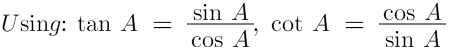=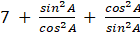=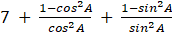=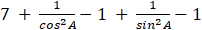=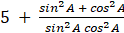= 5 + 1/(sin2 A cos2 A) …(ii)From equation (i) & (ii),LHS = RHSHence, proved.Question 10.Simplify (1 – cosθ) (1 + cosθ) (1 + cot2θ)Answer:We have(1 – cos θ)(1 + cos θ)(1 + cot2 θ) = [(1 – cos θ)(1 + cos θ)](1 + cot2 θ)⇒ (1 – cos θ)(1 + cos θ)(1 + cot2 θ) = (1 – cos2 θ)(1 + cot2 θ) [∵, (a + b)(a – b) = a2 – b2]⇒ (1 – cos θ)(1 + cos θ)(1 + cot2 θ) = sin2 θ × (1 + cos2 θ/sin2 θ) [∵, (1 – cos2 θ) = sin2 θ & cot2 θ = cos2 θ/sin2 θ]⇒ (1 – cos θ)(1 + cos θ)(1 + cot2 θ) = sin2 θ × (sin2 θ + cos2 θ)/sin2 θ⇒ (1 – cos θ)(1 + cos θ)(1 + cot2 θ) = sin2 θ + cos2 θ⇒ (1 – cos θ)(1 + cos θ)(1 + cot2 θ) = 1 [∵, sin2 θ + cos2 θ = 1]Thus, (1 – cos θ)(1 + cos θ)(1 + cot2 θ) = 1.Question 11.If secθ + tanθ = p, then what is the value of secθ – tanθ ?Answer:Given that, sec θ + tan θ = p.By trigonometric identity, we havesec2 θ – tan2 θ = 1So, sec2 θ – tan2 θ = 1⇒ (sec θ – tan θ) (sec θ + tan θ) = 1⇒ sec θ – tan θ = 1/(sec θ + tan θ)⇒ sec θ – tan θ = 1/p [given]Hence, sec θ – tan θ = 1/p.Question 12.If cosesθ + cotθ = k then prove that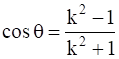Answer:Given that, cosec θ + cot θ = k⇒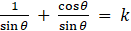[∵, cosec θ = 1/sin θ & cot θ = cos θ/sin θ]⇒ (1 + cos θ)/sin θ = k⇒ 1 + cos θ = k sin θSquaring both sides, we get(1 + cos θ)2 = (k sin θ)2⇒ (1 + cos θ)2 = k2 sin2 θ⇒ (1 + cos θ)2 = k2 (1 – cos2 θ) [∵, sin2 θ + cos2 θ = 1 ⇒ sin2 θ = 1 – cos2 θ]⇒ (1 + cos θ)2 = k2 (1 – cos θ) (1 + cos θ) [∵, a2 – b2 = (a + b) (a – b)]⇒ 1 + cos θ = k2 (1 – cos θ)⇒ 1 + cos θ = k2 – k2 cos θ⇒ k2 cos θ + cos θ = k2 – 1⇒ cos θ (k2 + 1) = k2 – 1⇒ cos θ = (k2 – 1)/(k2 + 1)Thus, cos θ = (k2 – 1)/(k2 + 1).Hence, proved.

PDF FILE TO YOUR EMAIL IMMEDIATELY PURCHASE NOTES & PAPER SOLUTION. @ Rs. 50/- each (GST extra)

HINDI ENTIRE PAPER SOLUTION

MARATHI PAPER SOLUTION

SSC MATHS I PAPER SOLUTION

SSC MATHS II PAPER SOLUTION

SSC SCIENCE I PAPER SOLUTION

SSC SCIENCE II PAPER SOLUTION

SSC ENGLISH PAPER SOLUTION

SSC & HSC ENGLISH WRITING SKILL

HSC ACCOUNTS NOTES

HSC OCM NOTES

HSC ECONOMICS NOTES

HSC SECRETARIAL PRACTICE NOTES

# 2019 Board Paper Solution

HSC ENGLISH SET A 2019 21st February, 2019

HSC ENGLISH SET B 2019 21st February, 2019

HSC ENGLISH SET C 2019 21st February, 2019

HSC ENGLISH SET D 2019 21st February, 2019

SECRETARIAL PRACTICE (S.P) 2019 25th February, 2019

HSC XII PHYSICS 2019 25th February, 2019

CHEMISTRY XII HSC SOLUTION 27th, February, 2019

OCM PAPER SOLUTION 2019 27th, February, 2019

HSC MATHS PAPER SOLUTION COMMERCE, 2nd March, 2019

HSC MATHS PAPER SOLUTION SCIENCE 2nd, March, 2019

SSC ENGLISH STD 10 5TH MARCH, 2019.

HSC XII ACCOUNTS 2019 6th March, 2019

HSC XII BIOLOGY 2019 6TH March, 2019

HSC XII ECONOMICS 9Th March 2019

SSC Maths I March 2019 Solution 10th Standard11th, March, 2019

SSC MATHS II MARCH 2019 SOLUTION 10TH STD.13th March, 2019

SSC SCIENCE I MARCH 2019 SOLUTION 10TH STD. 15th March, 2019.

SSC SCIENCE II MARCH 2019 SOLUTION 10TH STD. 18th March, 2019.

SSC SOCIAL SCIENCE I MARCH 2019 SOLUTION20th March, 2019

SSC SOCIAL SCIENCE II MARCH 2019 SOLUTION, 22nd March, 2019

XII CBSE - BOARD - MARCH - 2019 ENGLISH - QP + SOLUTIONS, 2nd March, 2019

# HSCMaharashtraBoardPapers2020

(Std 12th English Medium)

HSC ECONOMICS MARCH 2020

HSC OCM MARCH 2020

HSC ACCOUNTS MARCH 2020

HSC S.P. MARCH 2020

HSC ENGLISH MARCH 2020

HSC HINDI MARCH 2020

HSC MARATHI MARCH 2020

HSC MATHS MARCH 2020

# SSCMaharashtraBoardPapers2020

(Std 10th English Medium)

English MARCH 2020

HindI MARCH 2020

Hindi (Composite) MARCH 2020

Marathi MARCH 2020

Mathematics (Paper 1) MARCH 2020

Mathematics (Paper 2) MARCH 2020

Sanskrit MARCH 2020

Sanskrit (Composite) MARCH 2020

Science (Paper 1) MARCH 2020

Science (Paper 2)

Geography Model Set 1 2020-2021

MUST REMEMBER THINGS on the day of Exam

Are you prepared? for English Grammar in Board Exam.

Paper Presentation In Board Exam

How to Score Good Marks in SSC Board Exams

Tips To Score More Than 90% Marks In 12th Board Exam

How to write English exams?

How to prepare for board exam when less time is left

How to memorise what you learn for board exam

No. 1 Simple Hack, you can try out, in preparing for Board Exam

How to Study for CBSE Class 10 Board Exams Subject Wise Tips?

JEE Main 2020 Registration Process – Exam Pattern & Important Dates

NEET UG 2020 Registration Process Exam Pattern & Important Dates

How can One Prepare for two Competitive Exams at the same time?

8 Proven Tips to Handle Anxiety before Exams!